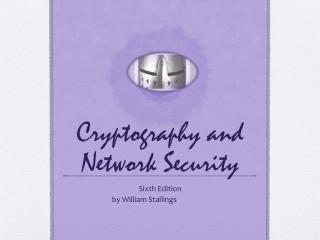# Cryptography and Network Security - PowerPoint PPT PresentationDownload PresentationCryptography and Network Security

Cryptography and Network SecurityDownload Presentation## Cryptography and Network Security

- - - - - - - - - - - - - - - - - - - - - - - - - - - E N D - - - - - - - - - - - - - - - - - - - - - - - - - - -
##### Presentation Transcript

1. Cryptography and Network Security Sixth Edition by William Stallings

2. Chapter 8 More Number Theory

3. The Devil said to Daniel Webster: “Set me a task I can’t carry out, and I’ll give you anything in the world you ask for.” Daniel Webster: “Fair enough. Prove that for n greater than 2, the equation an + bn = cn has no non-trivial solution in the integers.” They agreed on a three-day period for the labor, and the Devil disappeared. At the end of three days, the Devil presented himself, haggard, jumpy, biting his lip. Daniel Webster said to him, “Well, how did you do at my task? Did you prove the theorem?” “Eh? No … no, I haven’t proved it.” “Then I can have whatever I ask for? Money? The Presidency?” “What? Oh, that—of course. But listen! If we could just prove the following two lemmas—” —The Mathematical Magpie, Clifton Fadiman

4. Prime Numbers • Prime numbers only have divisors of 1 and itself • They cannot be written as a product of other numbers • Prime numbers are central to number theory • Any integer a > 1 can be factored in a unique way as a = p1a1 * p2a2 * . . . * pp1a1 where p1 < p2 < . . . < pt are prime numbers and where each ai is a positive integer • This is known as the fundamental theorem of arithmetic

5. Table 8.1 Primes Under 2000

6. Fermat's Theorem • States the following: • If p is prime and a is a positive integer not divisible by p then ap-1 = 1 (mod p) • Sometimes referred to as Fermat’s Little Theorem • An alternate form is: • If p is prime and a is a positive integer then ap = a (mod p) • Plays an important role in public-key cryptography

7. Table 8.2Some Values of Euler’s Totient Function ø(n)

8. Euler's Theorem • States that for every a and n that are relatively prime: aø(n) = 1(mod n) • An alternative form is: aø(n)+1 = a(mod n) • Plays an important role in public-key cryptography

9. Miller-Rabin Algorithm • Typically used to test a large number for primality • Algorithm is: TEST (n)

10. Deterministic Primality Algorithm • Prior to 2002 there was no known method of efficiently proving the primality of very large numbers • All of the algorithms in use produced a probabilistic result • In 2002 Agrawal, Kayal, and Saxena developed an algorithm that efficiently determines whether a given large number is prime • Known as the AKS algorithm • Does not appear to be as efficient as the Miller-Rabin algorithm

11. Chinese Remainder Theorem (CRT) • Believed to have been discovered by the Chinese mathematician Sun-Tsu in around 100 A.D. • One of the most useful results of number theory • Says it is possible to reconstruct integers in a certain range from their residues modulo a set of pairwise relatively prime moduli • Can be stated in several ways

12. Table 8.3 Powers of Integers, Modulo 19

13. Summary • Prime numbers • Fermat’s Theorem • Euler’s totient function • Euler’s Theorem • Testing for primality • Miller-Rabin algorithm • A deterministic primality algorithm • Distribution of primes • The Chinese Remainder Theorem • Discrete logarithms • Powers of an integer, modulo n • Logarithms for modular arithmetic • Calculation of discrete logarithms